4

# Level Based Measures

A Level Based Measure is a measure evaluated based on one or more predefined levels of aggregation (i.e. grouping dimension). Starting with Incorta Analytics 4.2, users can evaluate a measure for one or more aggregation levels in the same table Insight. This feature allows you to:

1. Evaluate measures grouped by different levels of aggregation in the same tabular Insight.
2. Analyze the contribution percentage of one aggregation level with respect to another (e.g. countries contribution percentage to the regional revenue).

To learn about this feature by example, let's consider a scenario where we want to analyze the sales revenue by the following aggregation levels:

• region
• subregion
• country

Prior to this feature, we would use Incorta's aggregated table as the visualization type, measuring the sales revenue grouped by region, sub-region, and country. In this case, we would have a column showing each country's revenue, since the country is the most granular level here.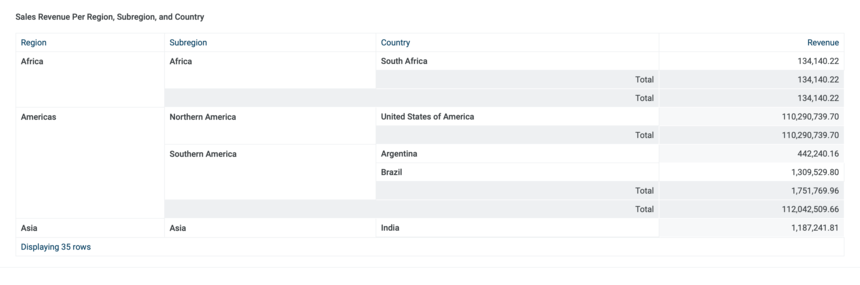Now, assume we want to add a column showing the sales regional revenue, then analyze each country's contribution to the regional revenue. In this case, we would need to add a level based measure (i.e. revenue based on the "region" level), then add a formula column calculating the country's contribution percentage with respect to the regional revenue.

Use the following steps for detailed instructions on how to use Level Based Measures:

1. Choose an "Aggregation Table" Insight from the visualization selector window, which appears when adding a new Insight.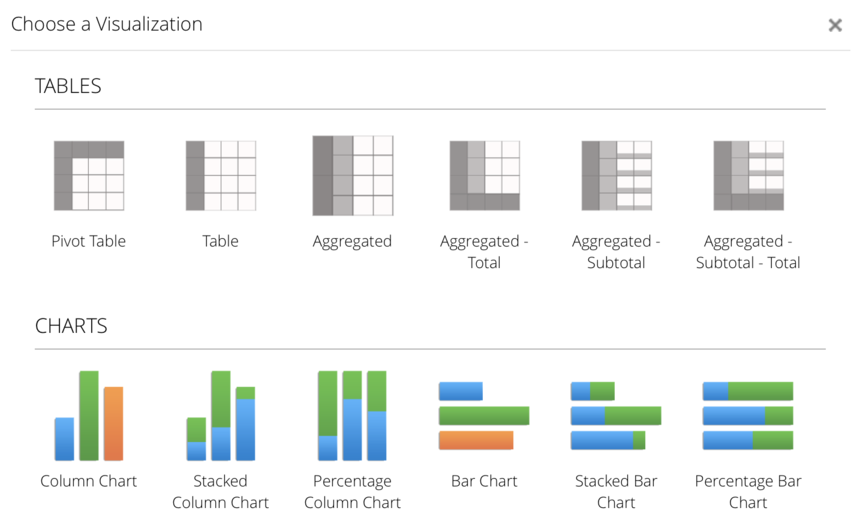2. Drag "Region", "Subregion", and "Country", respectively, from the source elements list on the left-hand side into the grouping dimension field.
3. Drag "Revenue" into the measure field.
4. Drag "New Formula" from the source elements list on the left-hand side into the "measure" field.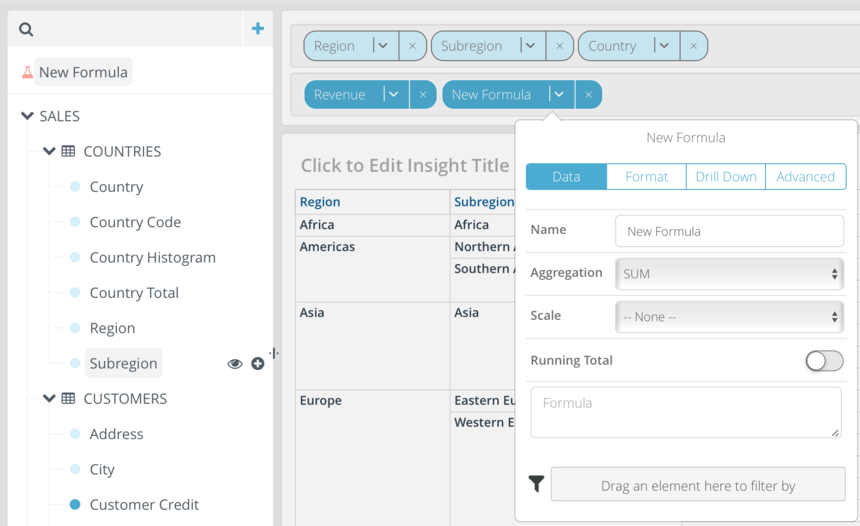5. Click in the "Formula" box to open the Formula Builder.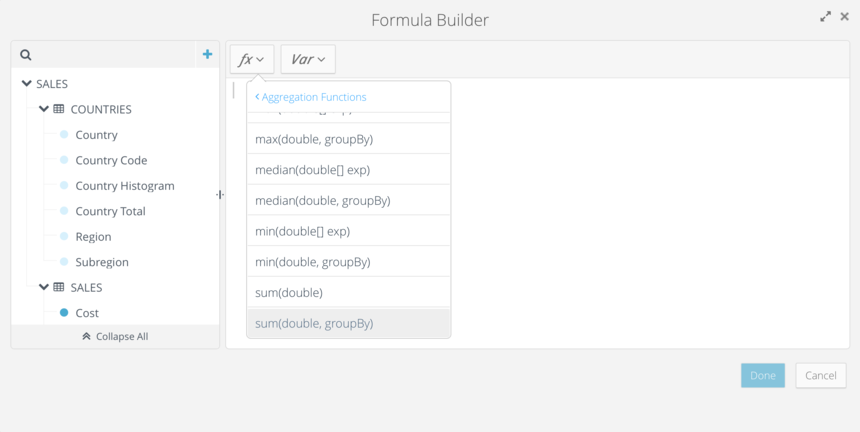6. Select "sum(double, groupBy)", under "Aggregation Functions" from the function (fx) menu.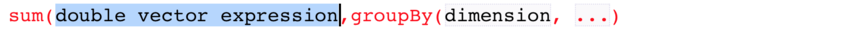7. Replace the "double vector expression" parameter with "Revenue" as the measure, from the source elements list on the left-hand side.
8. Replace the "dimension" parameter with "Region", from the source elements list on the left-hand side, to group by the region.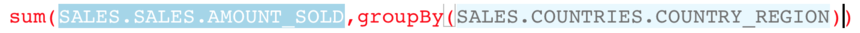9. Click Done. Notice the "Regional Revenue" column is now added to the Insight.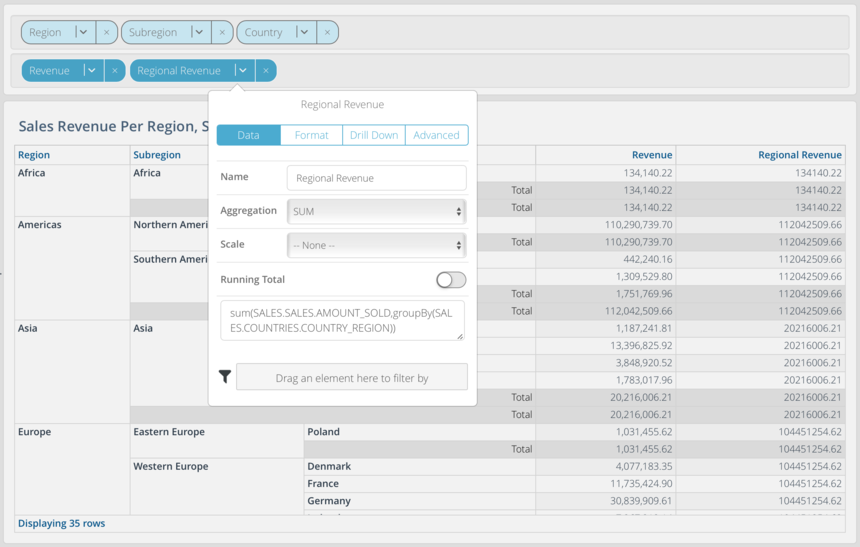10. Now, to add the percentage contribution column, repeat steps 4-5.
11. Select "sum(double)", under "Aggregation Functions" from the function (fx) menu. Then, divide it by "sum(double, groupBy)".
12. Replace all the double expressions with "Revenue", then replace the "dimension" parameter with "Region".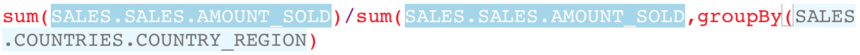13. Click Done. Notice the "Regional Revenue" column is now added to the Insight.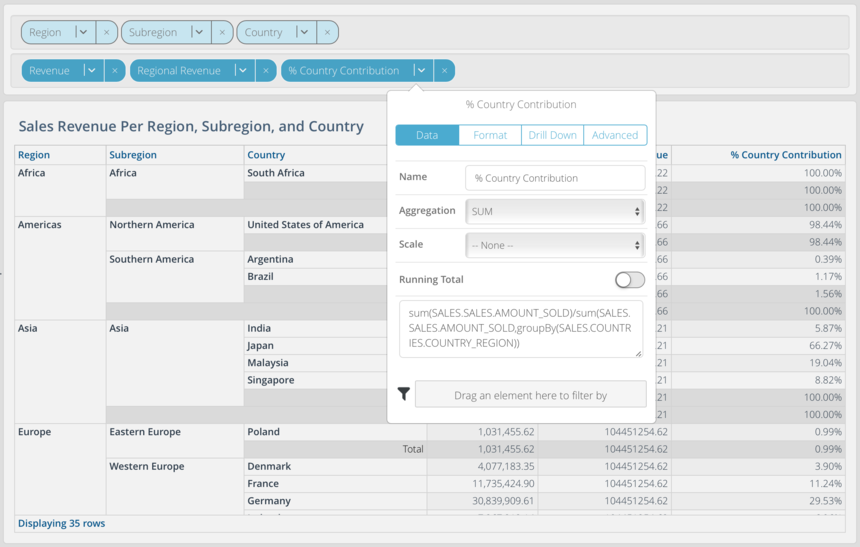Now let's redo the same analysis, swapping the regional revenue with the subregion revenue. Also, instead of calculating the country's contribution to the region, let's re-calculate the country's contribution to the subregion. It is important to note here that since the subregion is of lower hierarchical level than the region, we must group by all levels used in the Insight of higher hierarchy leading to the subregion level (i.e. region in this case).

To analyze the revenue for each subregion and calculate the contribution percentage of each country to the subregion, repeat steps 1-13 from the previous example, changing the following:

• the groupBy parameter to "Region" and add "Subregion" as a second grouping dimension.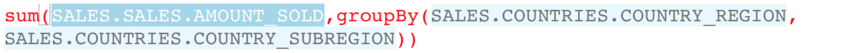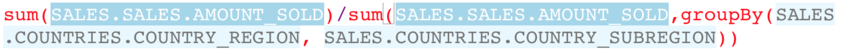The resulting Insight should show each country's contribution percentage with respect to its region.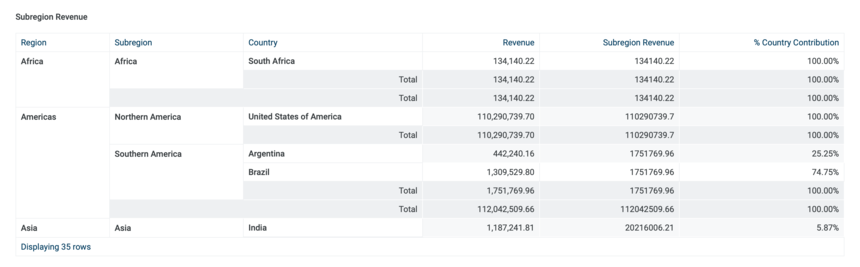Note:

When using a group-by dimension for a level based measure, it is important to include all the leading grouping dimensions in the Insight from the top down to the level based measure to be evaluated. In the example above, the revenue by sub-region included region and subregion in the group-by because the Insight had consisted of the same order in the grouping dimensions.

2replies Oldest first
• Oldest first